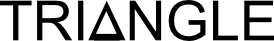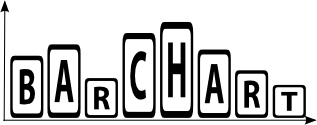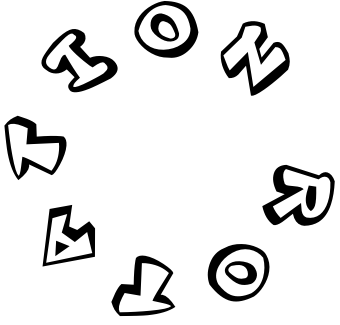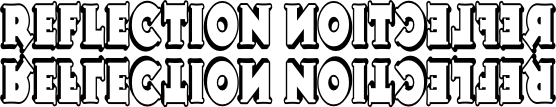# WordlesCan you think of some more?

#### Triangled HexagramArrange the twelve numbers in the triangles on the hexagram so that the numbers in each line of five triangles add up to the same total. So far this activity has been accessed 23 times and 3 people have earned a Transum Trophy for completing it.

## Wordles

#### Remember these mathematical words for their shape and movement.

There are many more mathematical vocabulary activities on Transum.org:

In-tents Story: Work out the mathematical words missing from the story about buying a tent.

Mathanagrams: The letters of mathematical words have been mixed up. Can you recognise them?

Maths Crossword: A short crossword containing mathematical words.

M-Words: Here are clues to some mathematical words all beginning with the letter M. Can you figure out what they are?

Mystery Numbers: If 16 O in a P stands for 16 ounces in a pound, what do you think these mystery numbers stand for?

Polygons: Match the shapes to the names of the polygons.

Vowelless: Vowels have been taken out of mathematical words. Can you recognise them?

Words in Digits: Write the numbers given in words as digits.

Do you have any comments? It is always useful to receive feedback and helps make this free resource even more useful for those learning Mathematics anywhere in the world. Click here to enter your comments.For All: